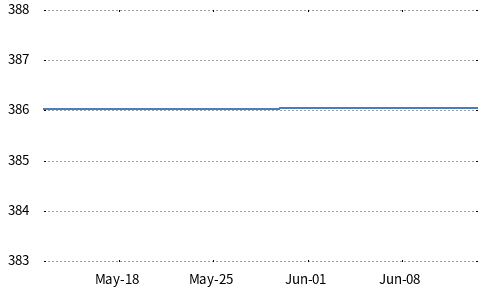Nikkei 225 Estimated Dividend Point Index
252.78
0.00 (0.00%)
May/20/2022(Close)
 | | | | | 1m |### Nikkei 225 70th anniversary website

Related News
Related Information
Overview
The Nikkei Stock Average Estimated Dividend Point Index is an index that accumulates dividends received from the companies when investors are supposed to hold the constituents of the Nikkei Stock Average (Nikkei 225) on a calendar year basis, i.e. January to December in a year. The index is calculated by substituting each estimated dividend per share into the formula of the Nikkei 225 on each ex-dividend date. Since the index value is adjusted when the estimate by Nikkei on the ex-dividend is different from the amount fixed, its final index value of the year is published at the beginning of April in the subsequent year after the all dividend payments of the constituents are fixed. The index is expressed with a year such as the Nikkei Stock Average Estimated Dividend Point Index (2019) since the index is calculated by accumulating the dividends every calendar year basis. The index value of the current year is displayed on this page.
Dividends to cover
Gross cash dividends whose ex-dividend dates are between January 1 and December 31, including special dividends and commemorative dividends
Calculation method
The index is calculated by substituting the received dividends of the constituents into the formula of the Nikkei 225. After adjusting each dividend by the price adjustment factor, the adjusted value shall be divided by the divisor on its ex-dividend date and accumulated. The index value is expressed with two decimal places in Japanese yen.
Timing to include
Dividends are accumulated in two steps, first on the day of ex-dividend, and then, adjusted it on the next date of fixing date when the estimate by Nikkei on the ex-dividend is different from the amount fixed. Dividends paid corresponding to the annual accounting period is fixed on the dates of the annual regular shareholder meetings. Dividends paid on the other dates is fixed on the dates of the publication by the companies.
Base date etc
The commencement date of the calculation was March 7th 2019, which had been retroactively calculated in the past to 1998. The index is calculated and published on the end-of-day basis at around 3pm on every business date.
•• Nikkei Inc. No reproduction without permission.